# Frank Solutions for Class 9 Maths Chapter 9 Indices

Frank Solutions for Class 9 Maths Chapter 9 Indices is an important study material for students from an exam point of view. These solutions are designed by subject-matter experts at BYJU’S who have vast conceptual knowledge. Students can practise these solutions on a daily basis, which will help them analyse the important questions that will appear in the final examination.

Chapter 9 solutions consist of problems based on Indices. The solutions are curated in such a way that students can grasp the concepts effortlessly. These solutions are available both online and offline modes and can be used as per their requirements. Students who aim to score good marks in the final exams can download Frank Solutions for Class 9 Maths Chapter 9 Indices PDF from the link available below and practise regularly.

## Frank Solutions for Class 9 Maths Chapter 9 Indices Download PDF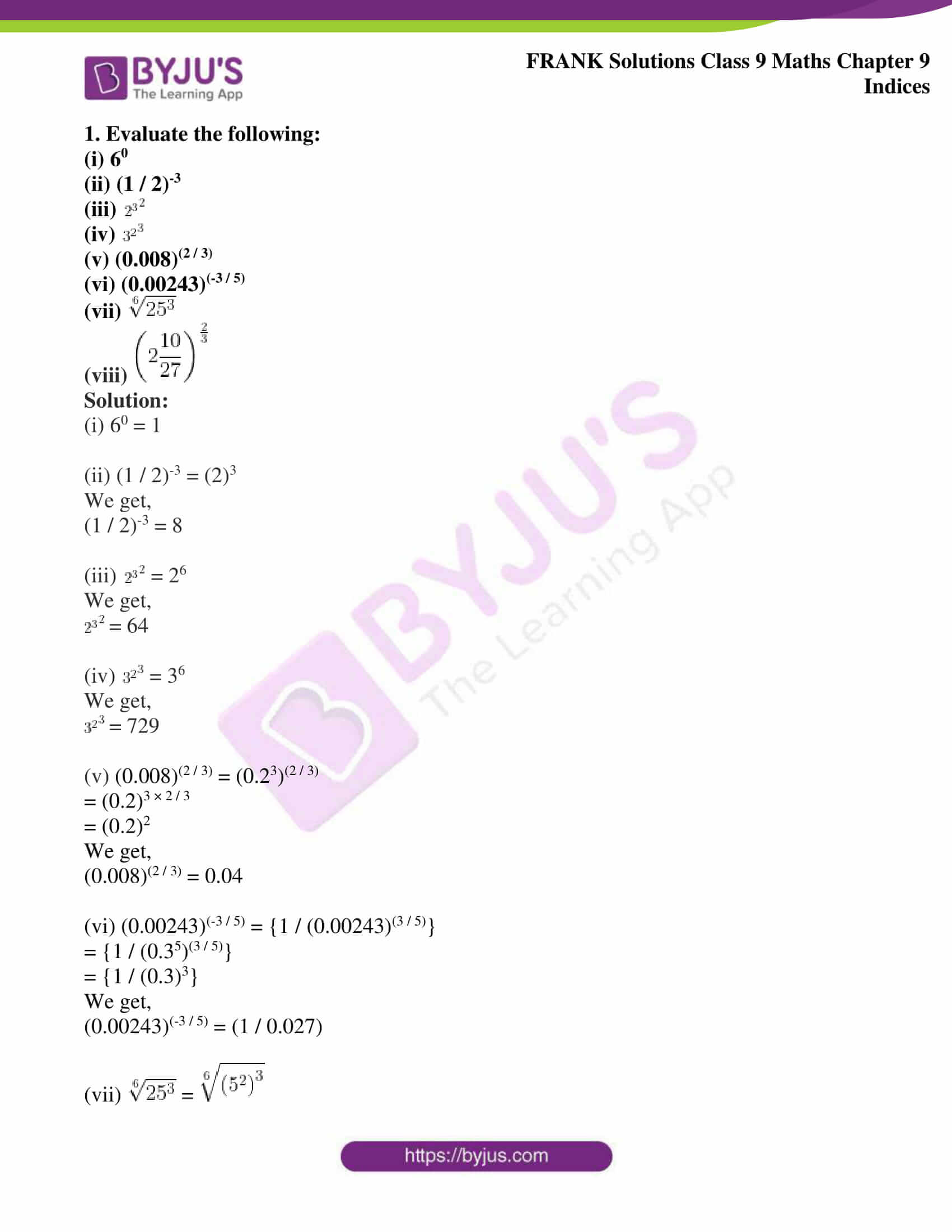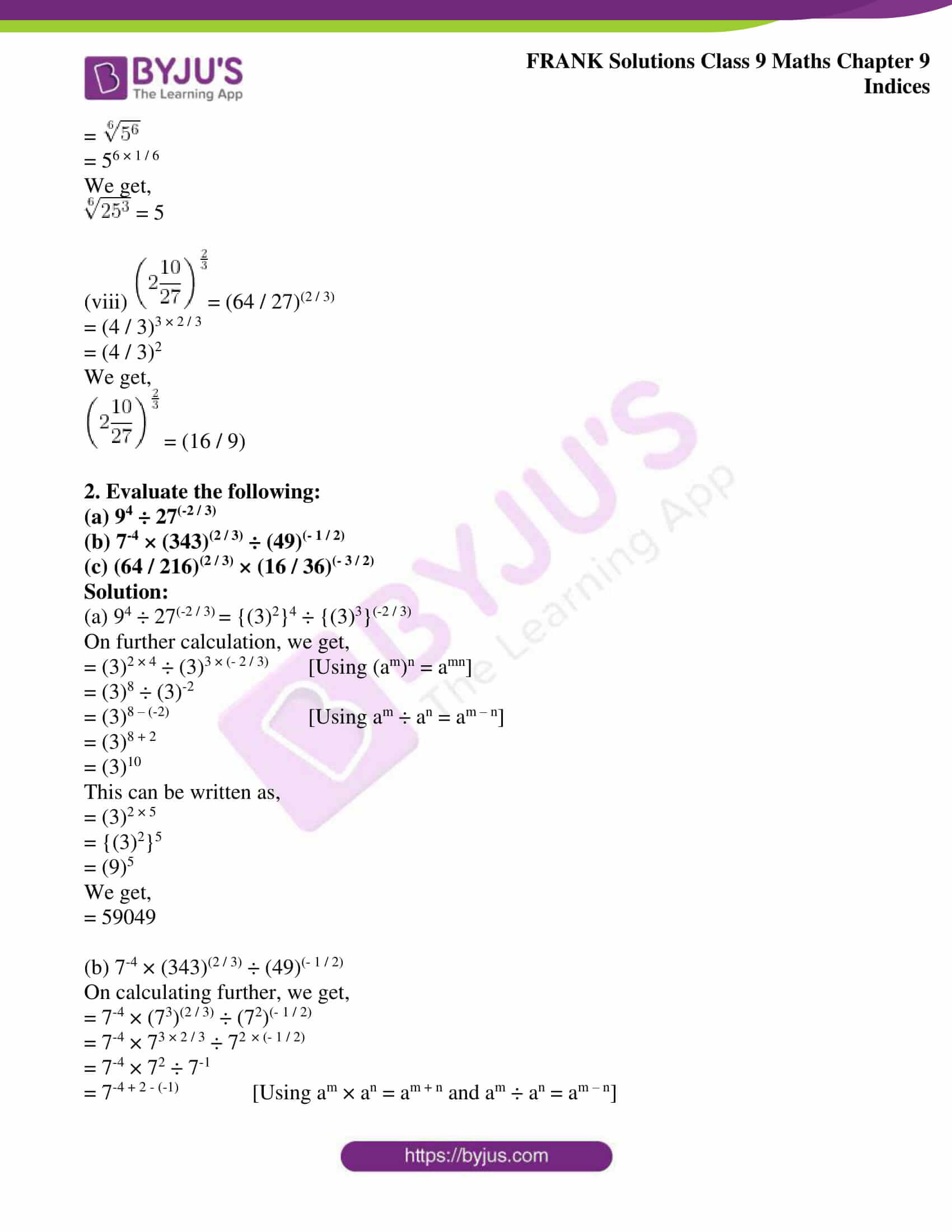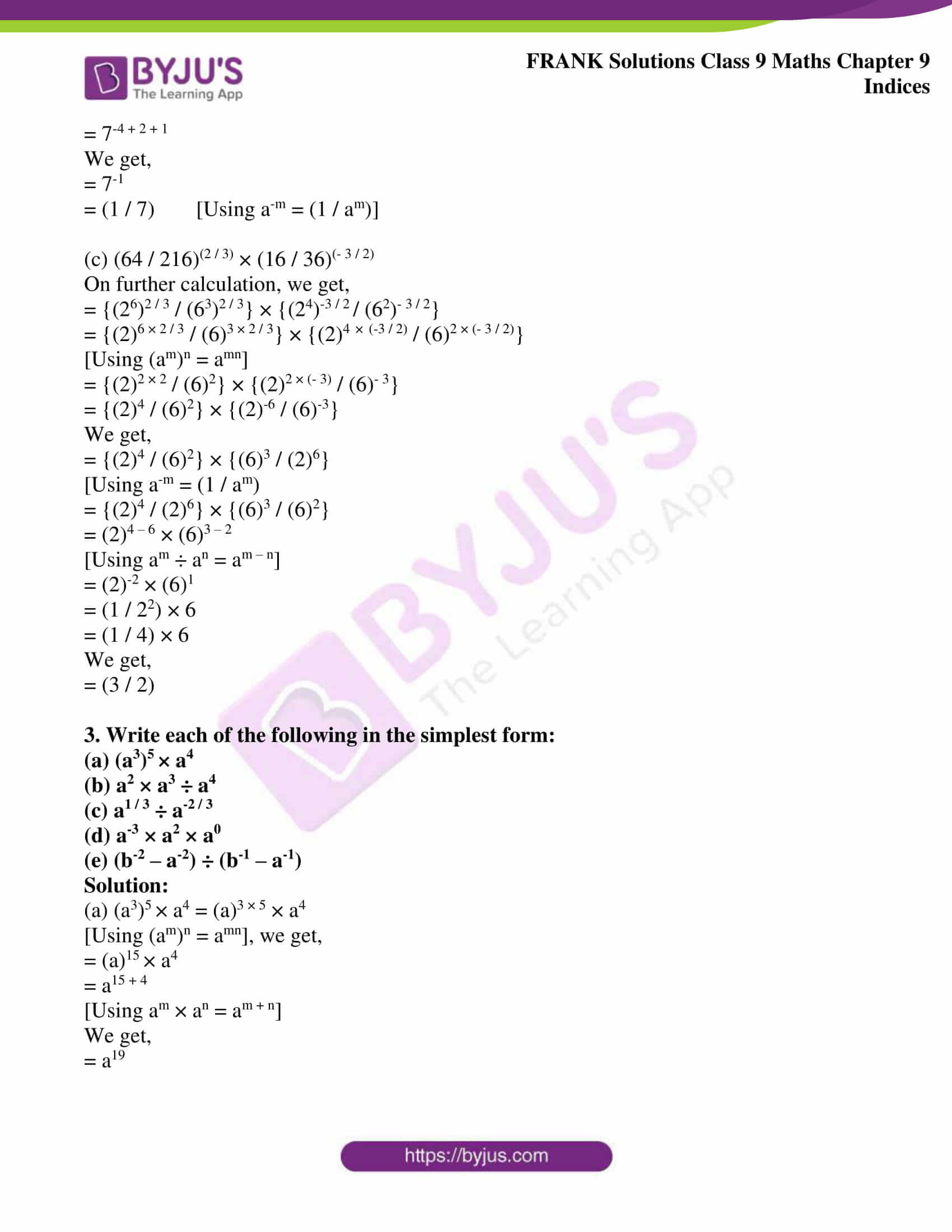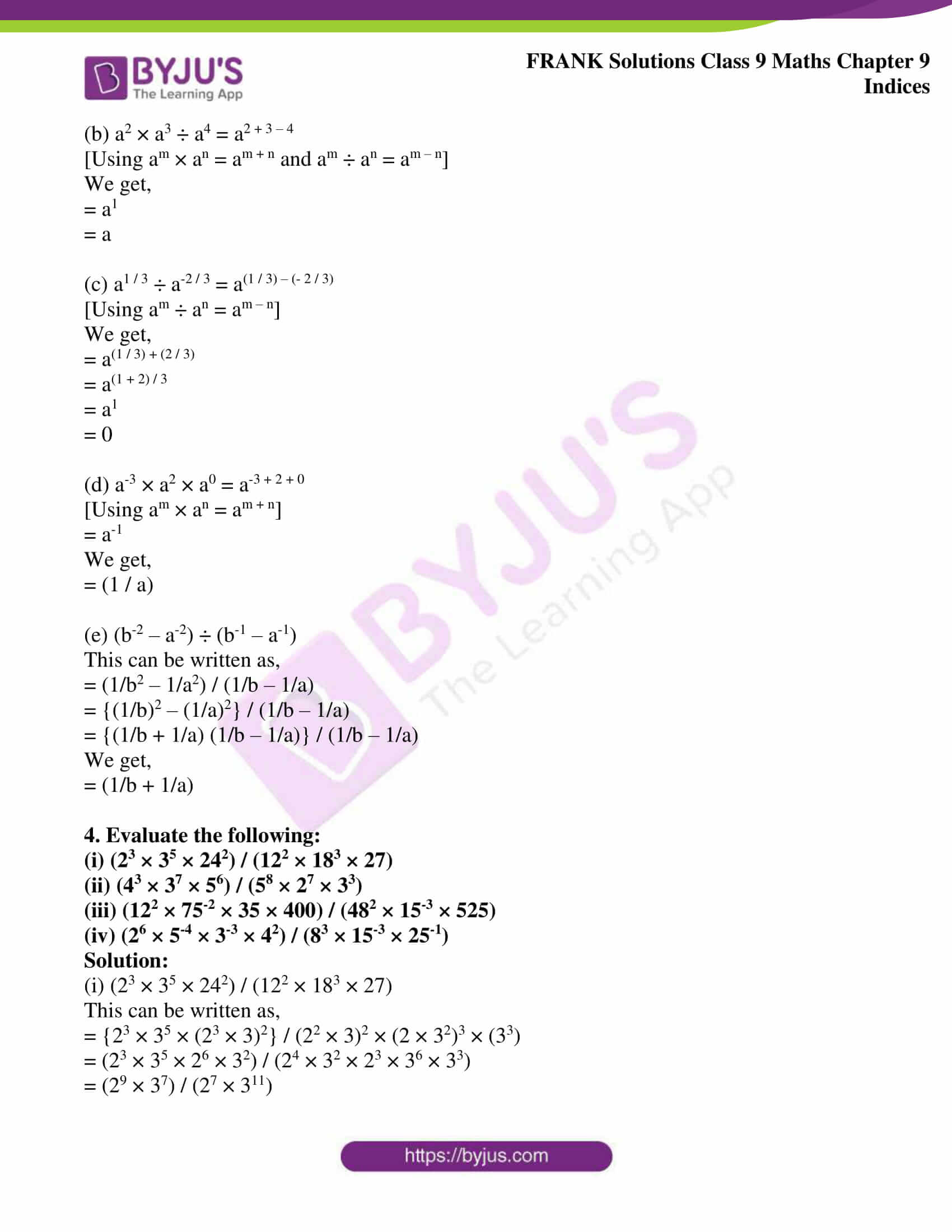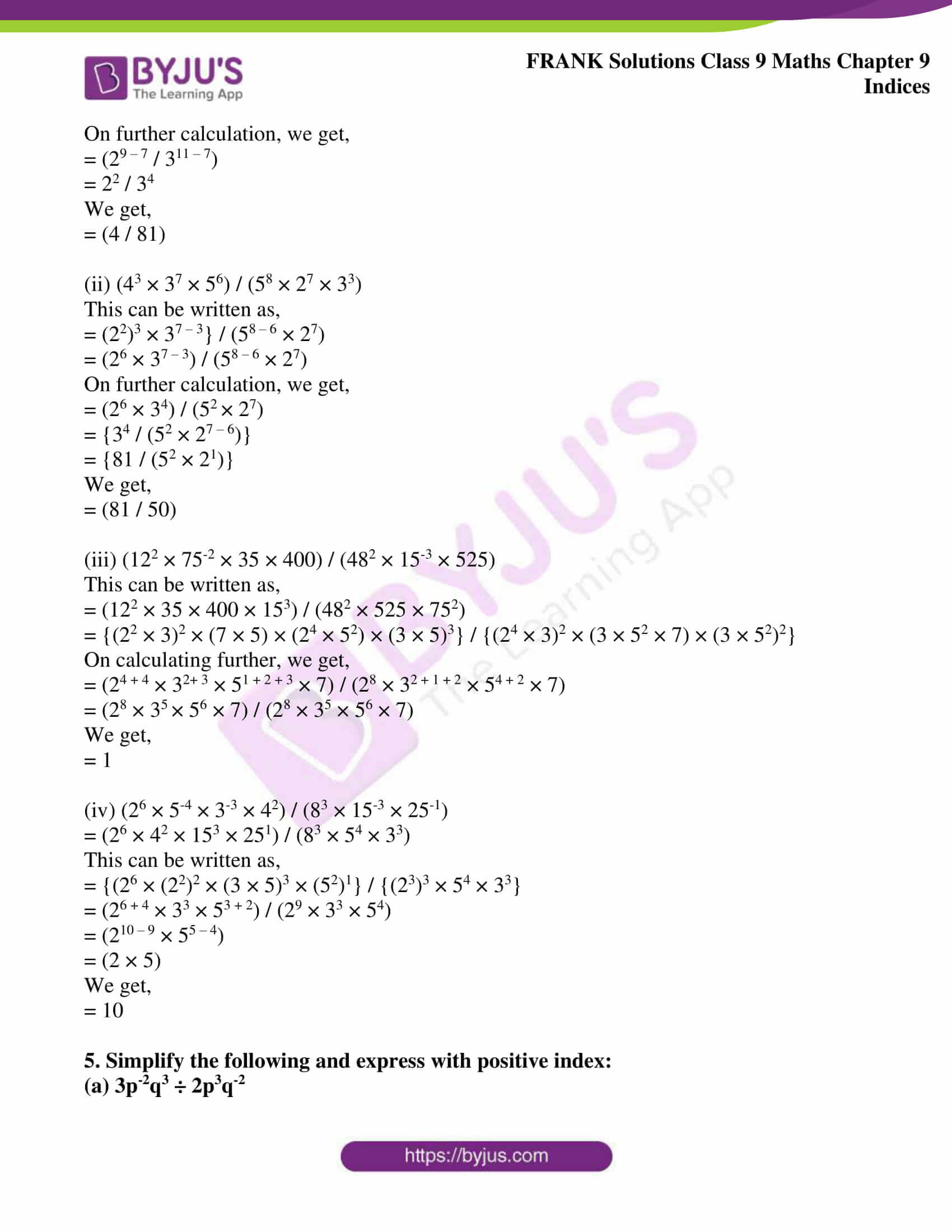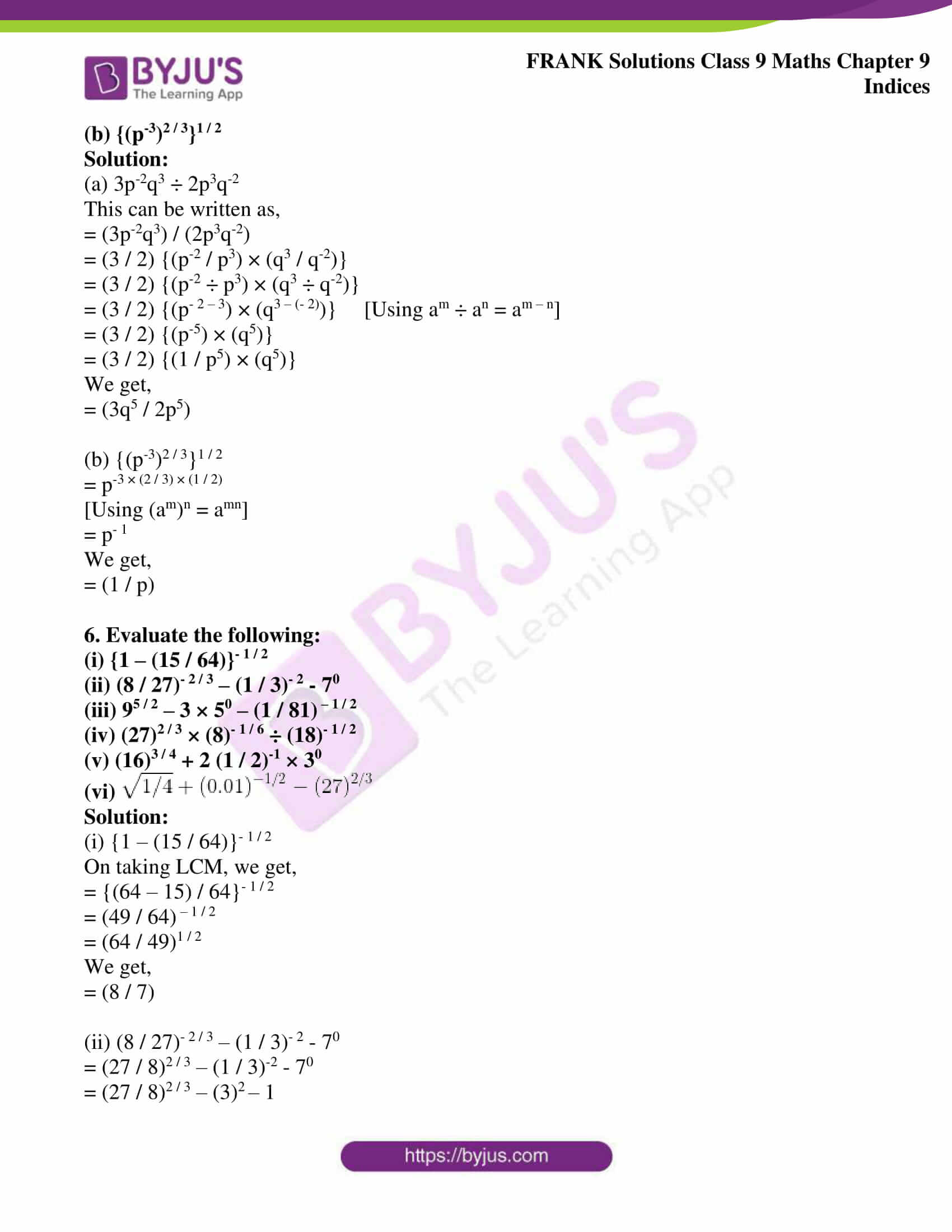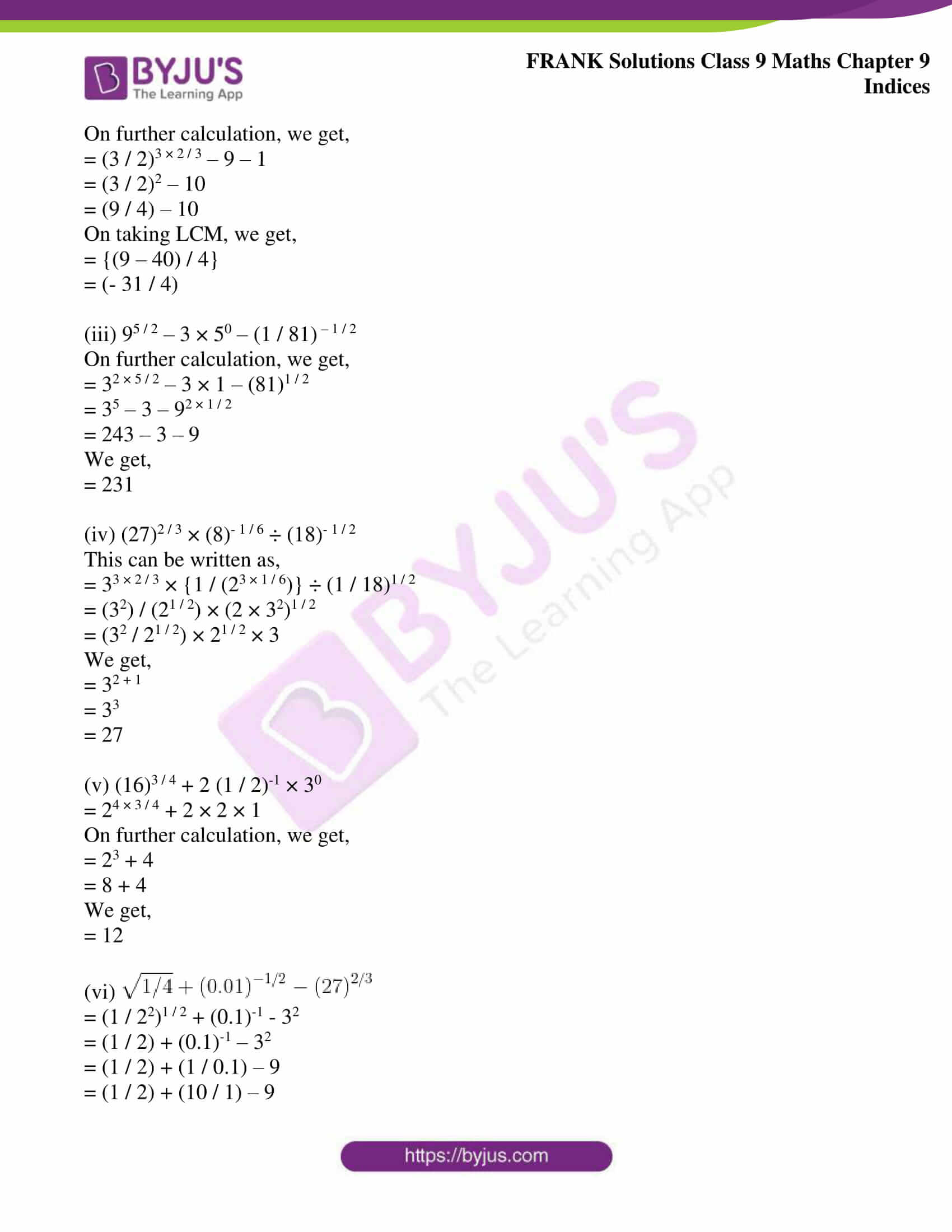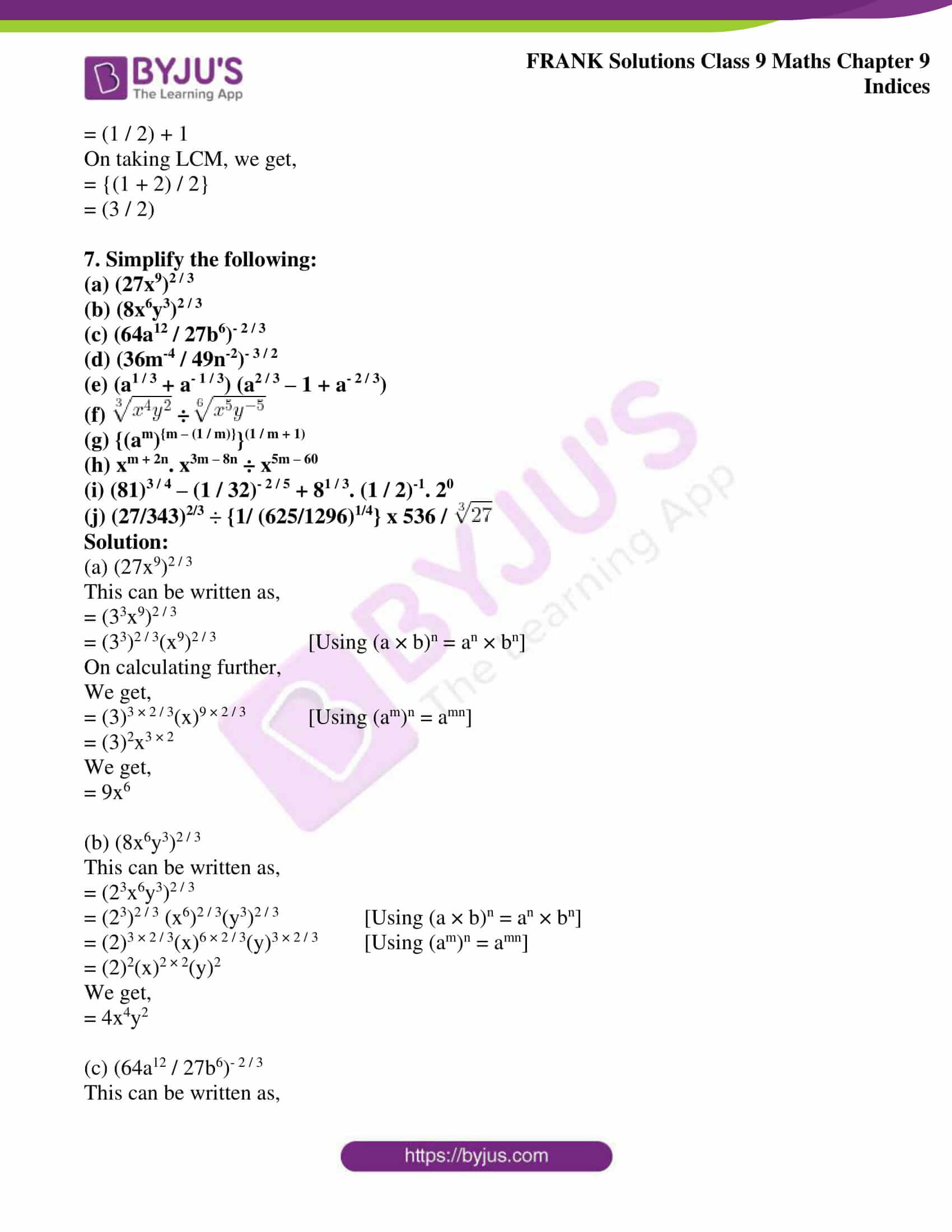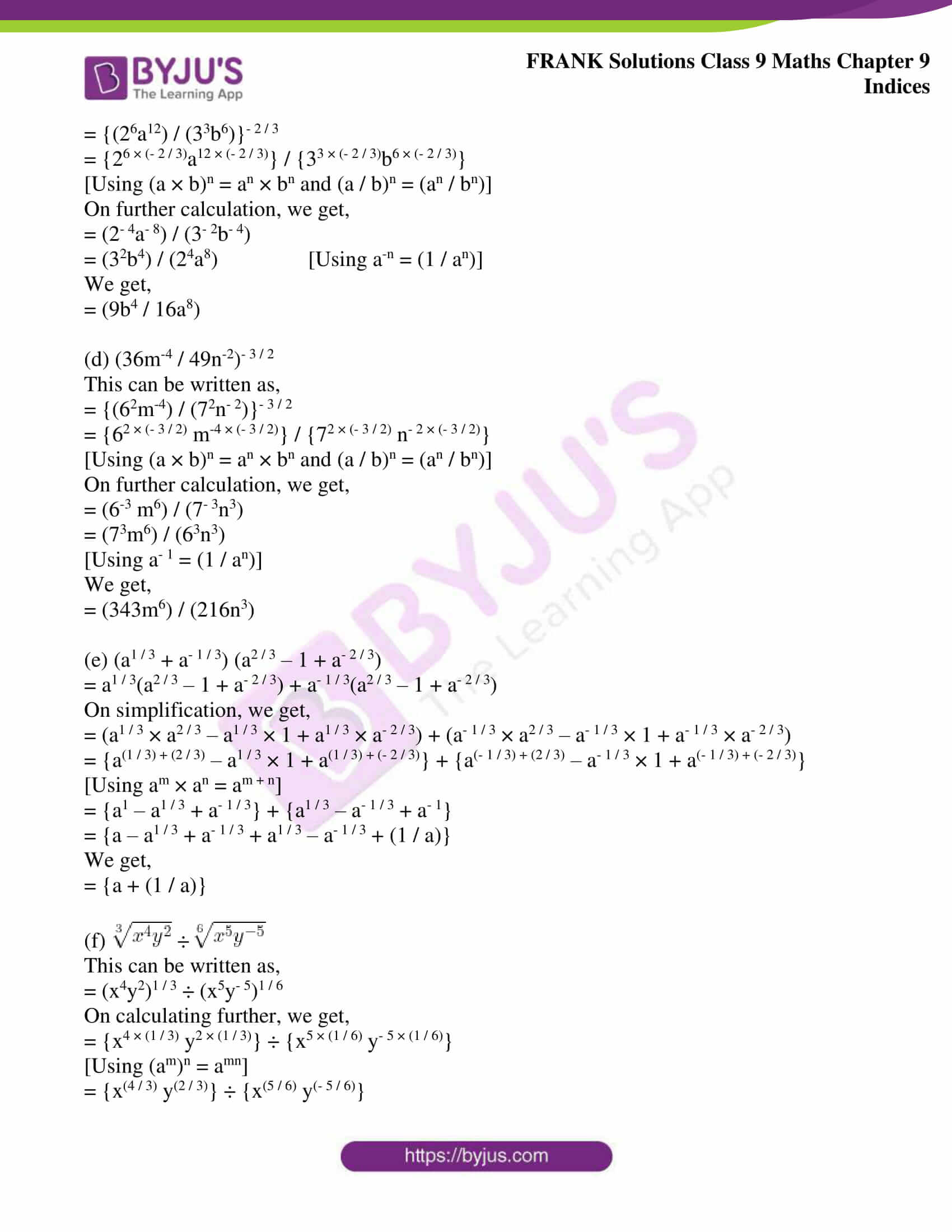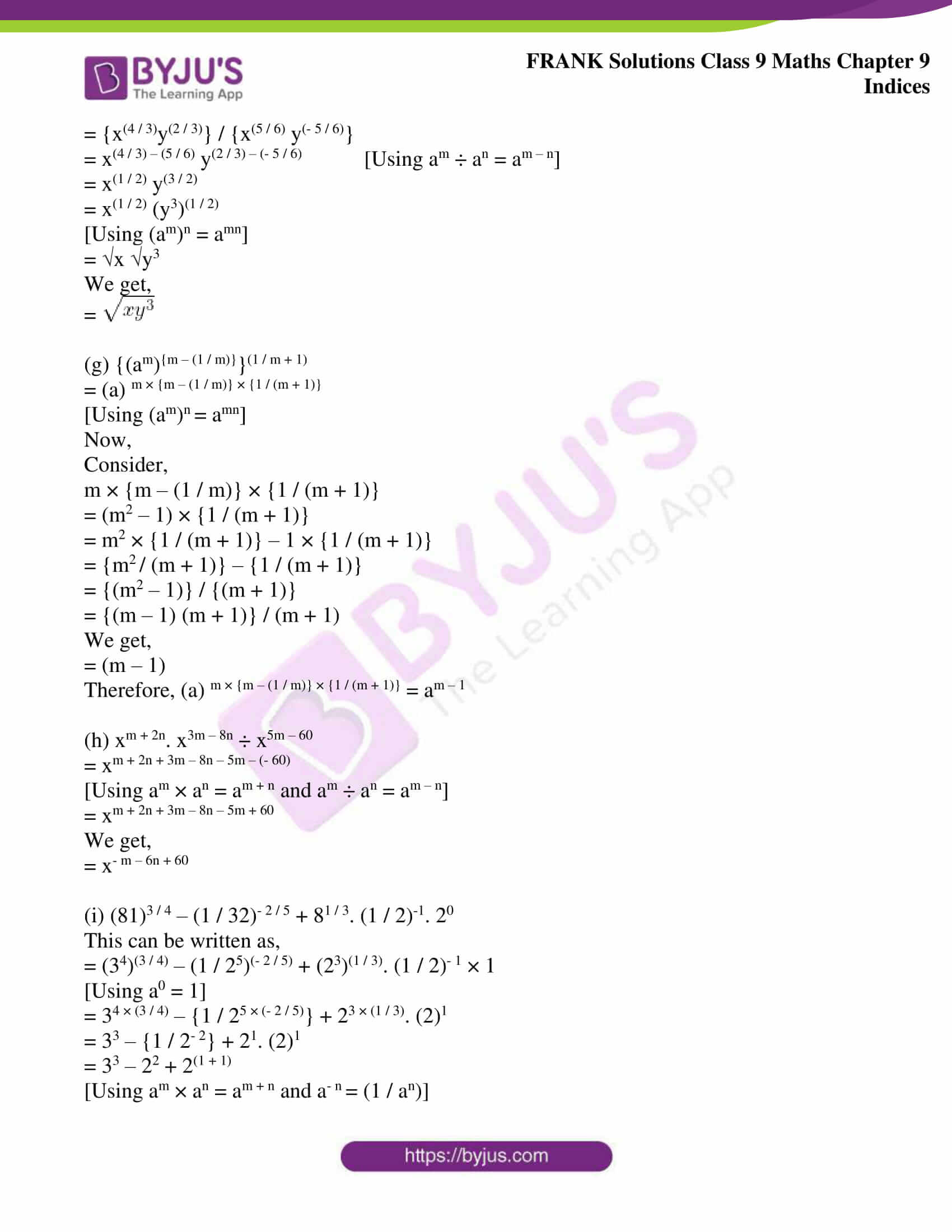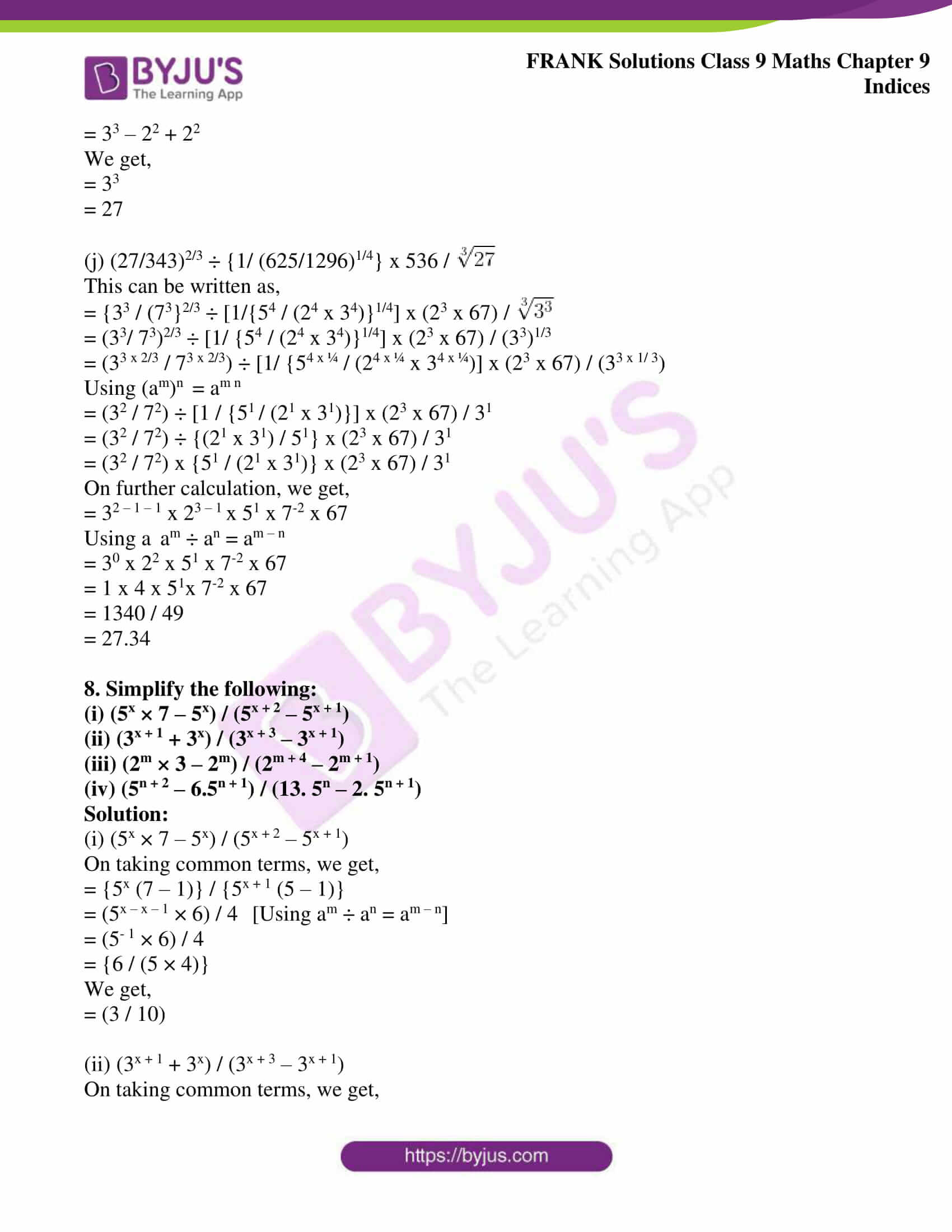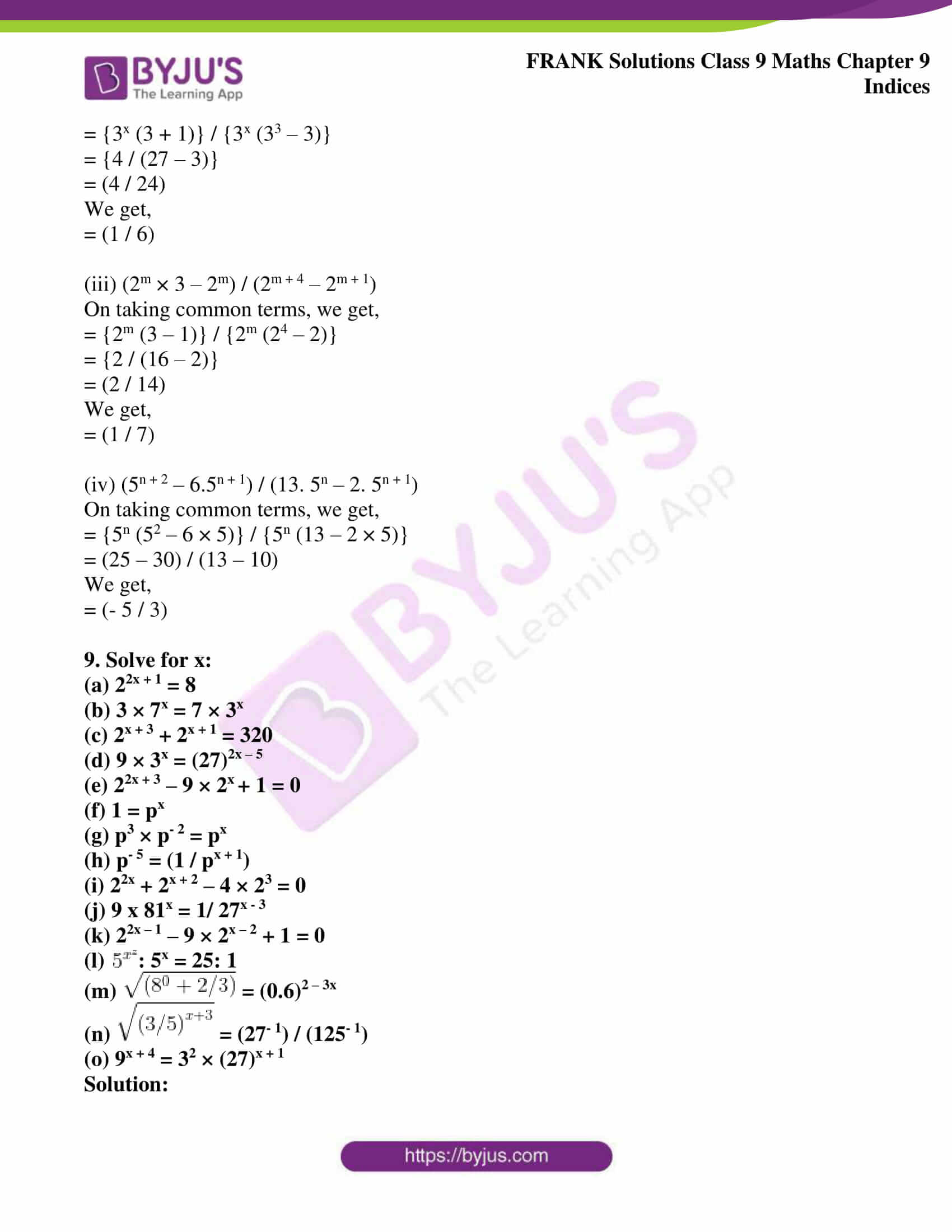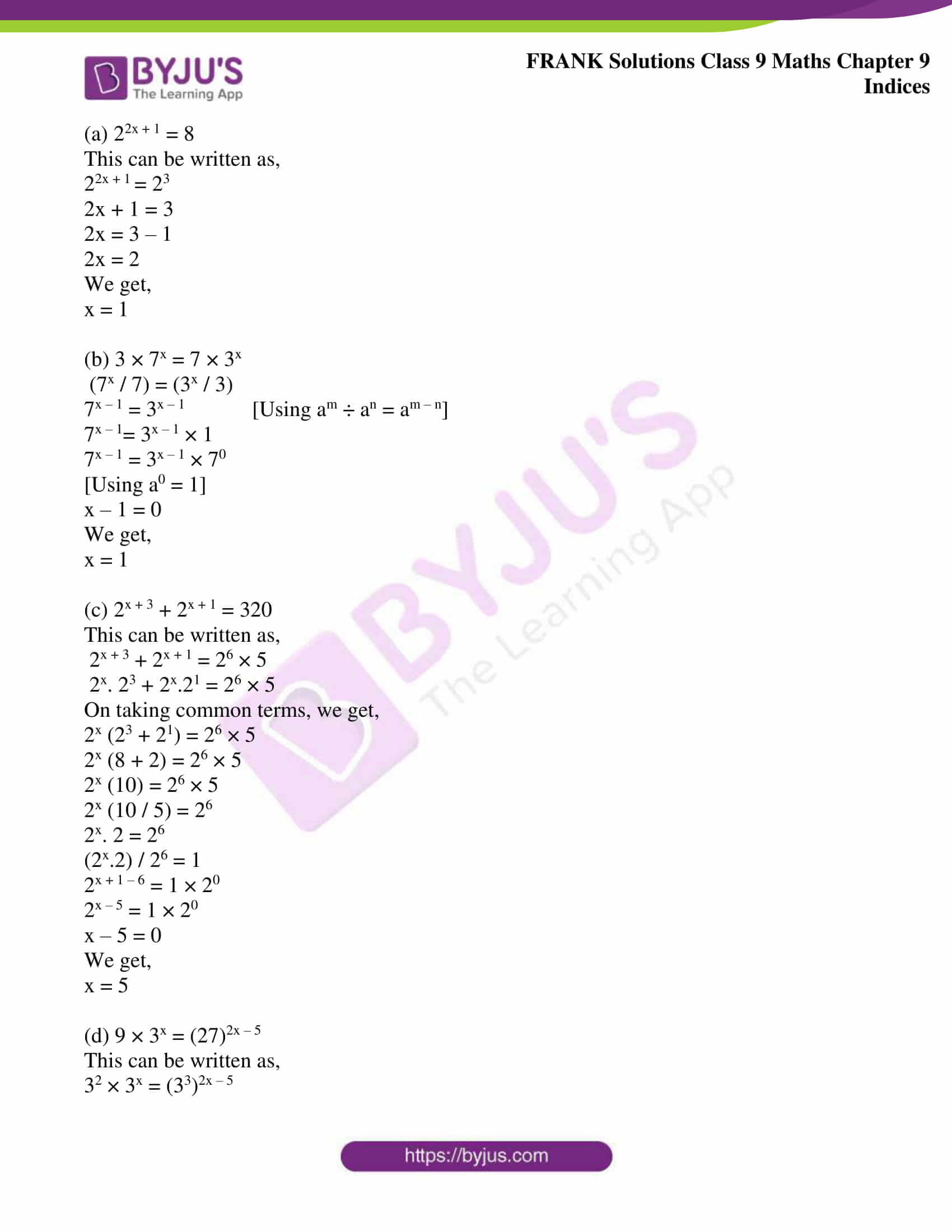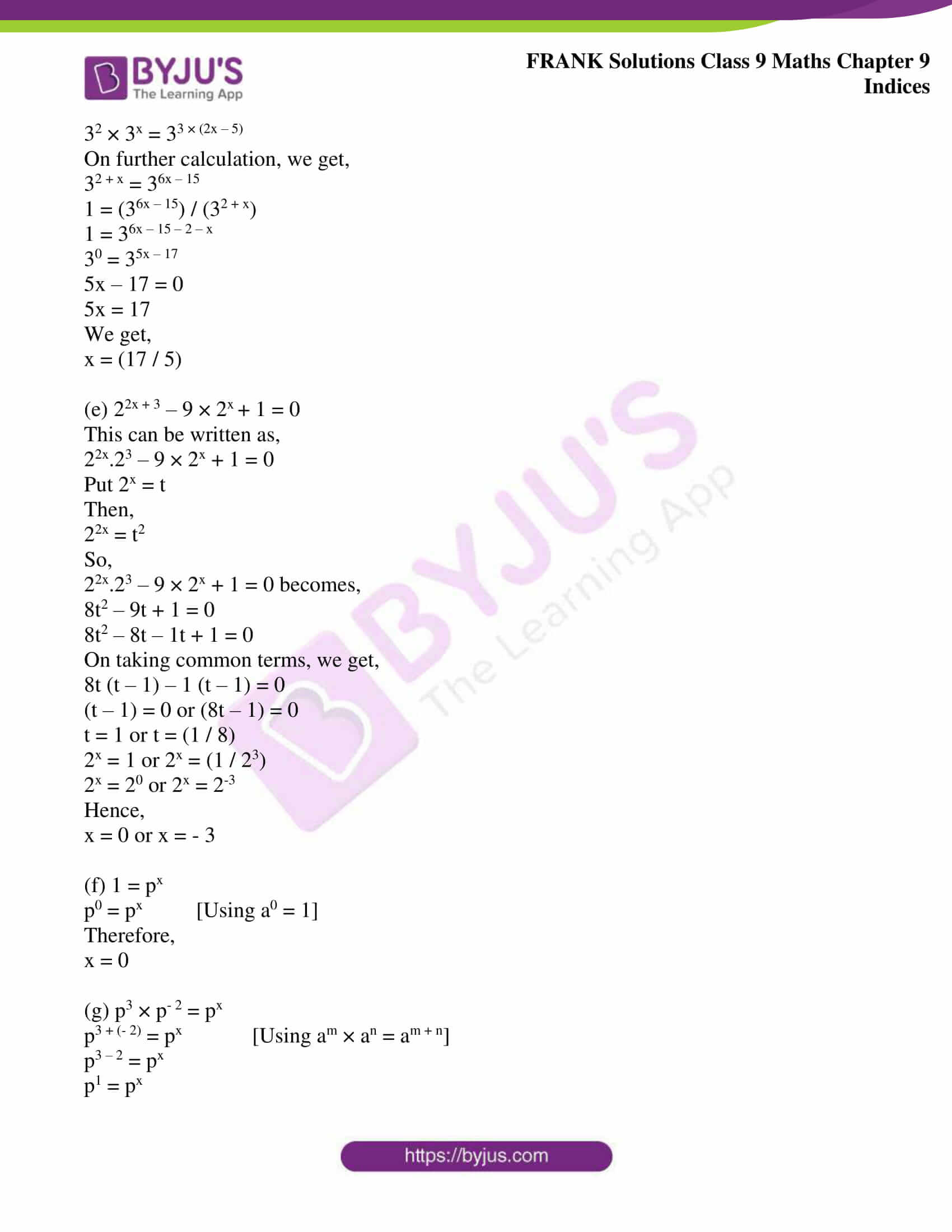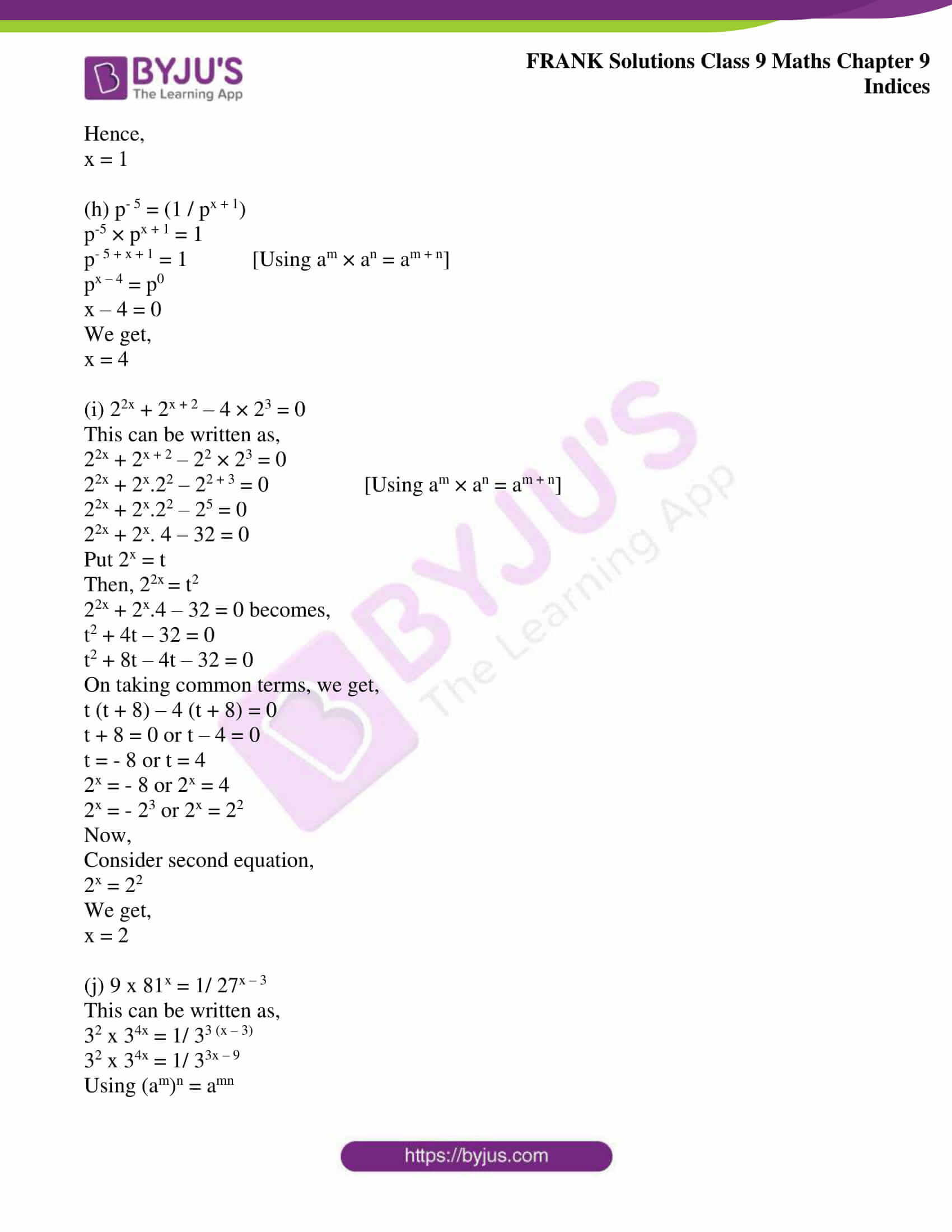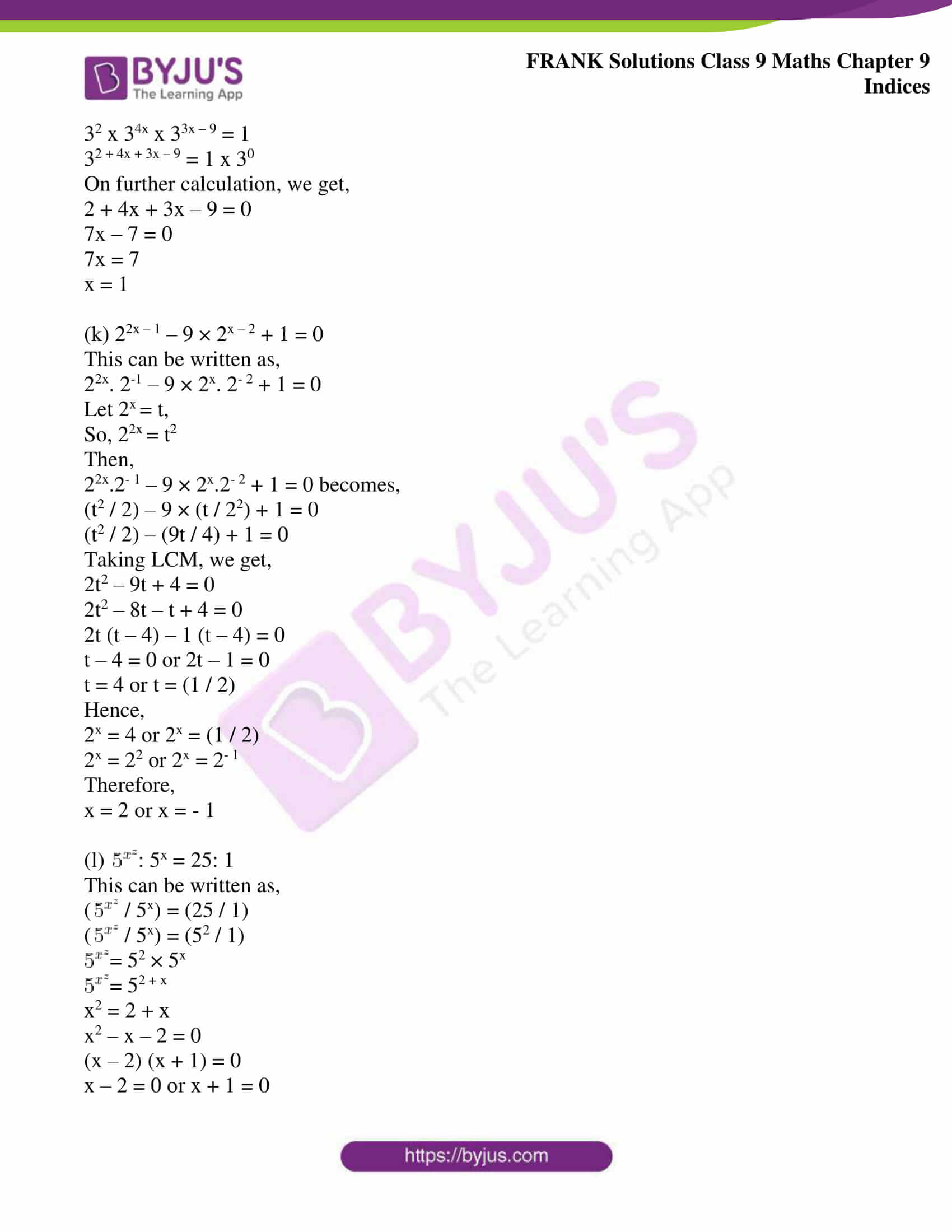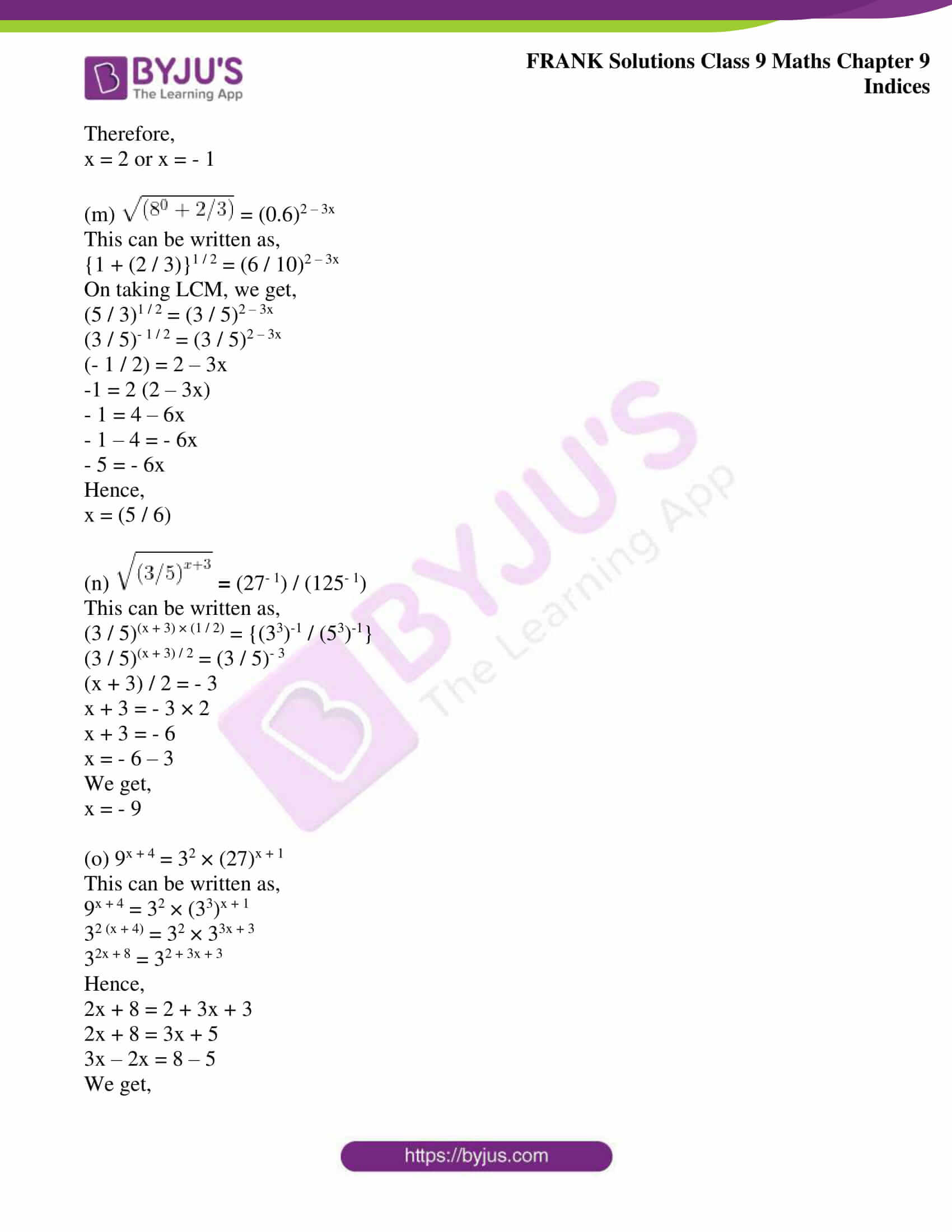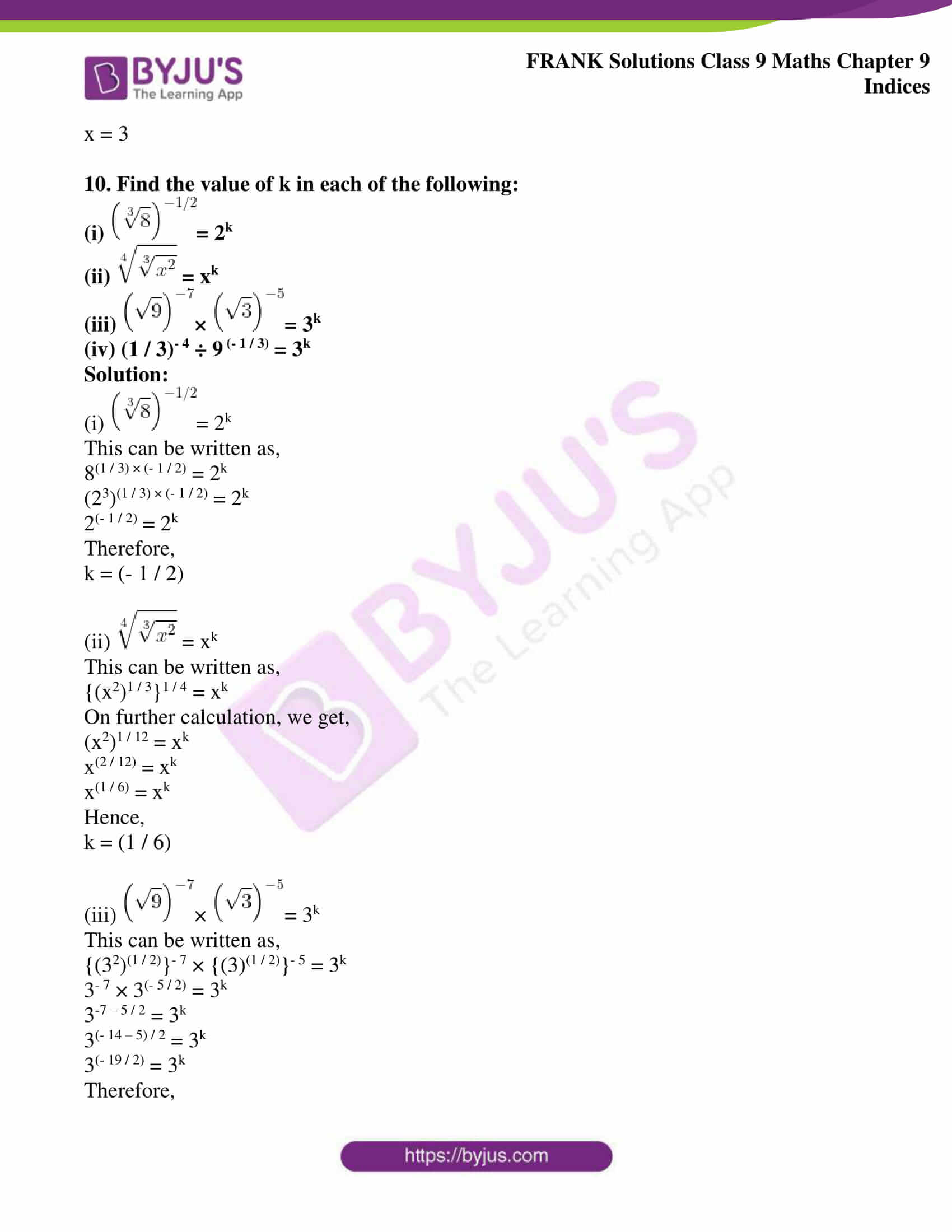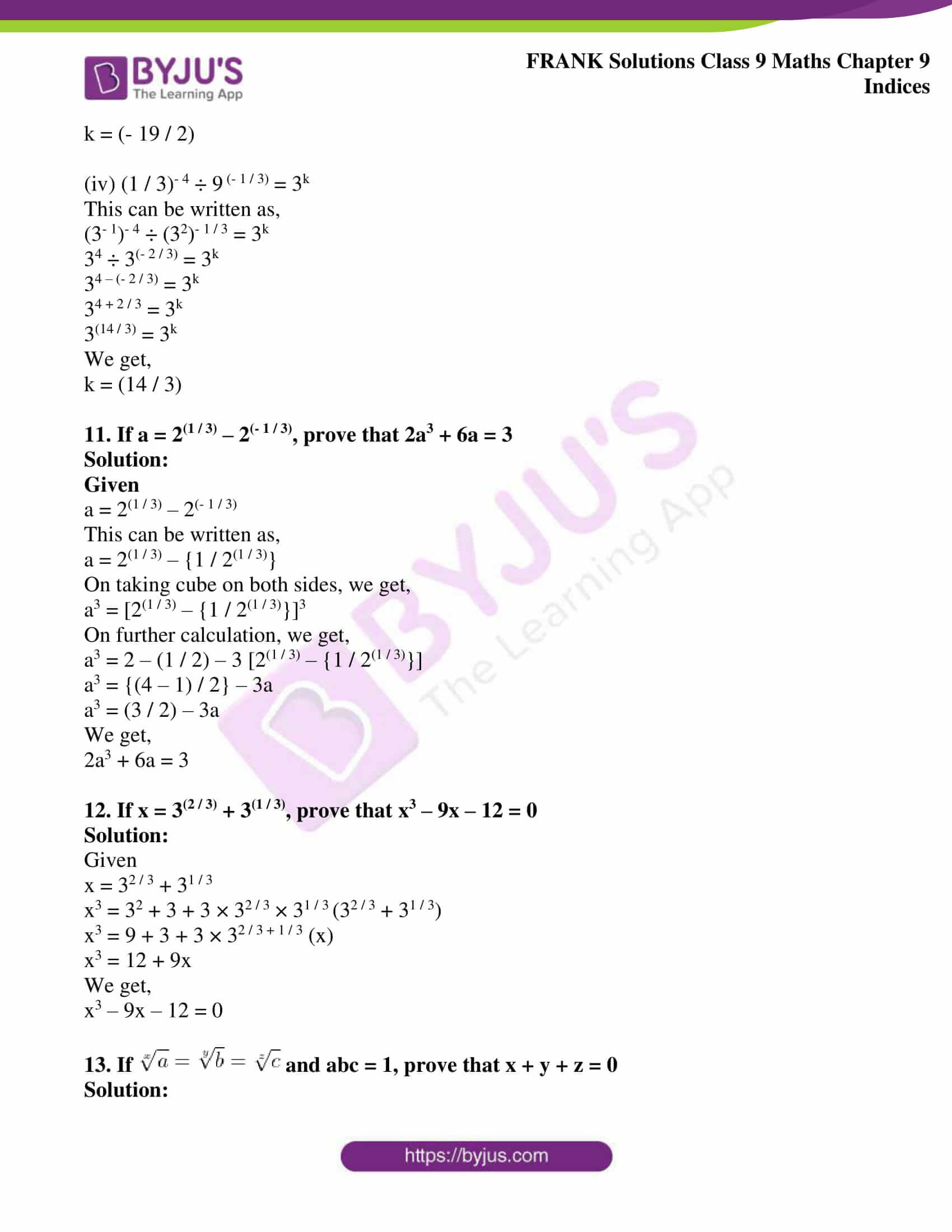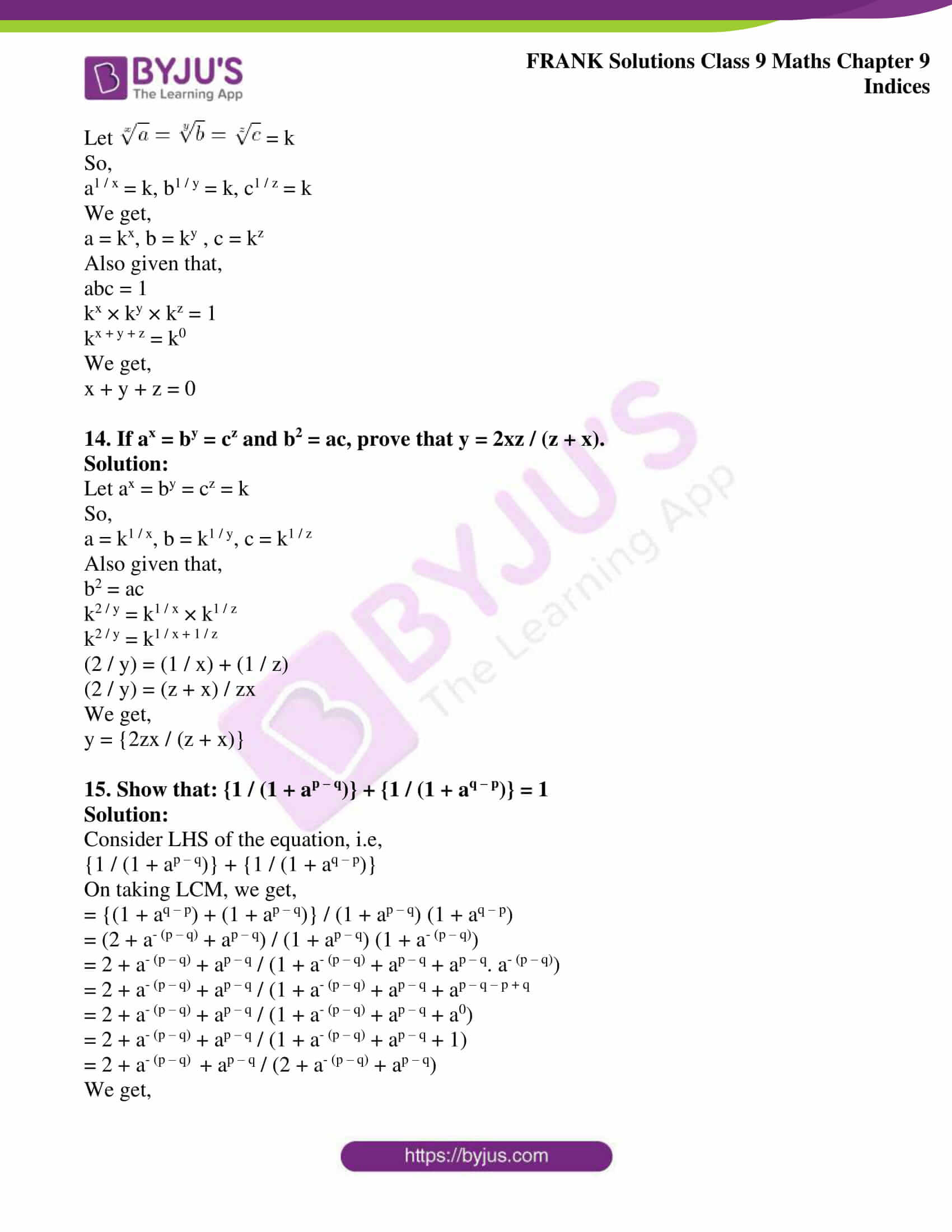## Access Frank Solutions for Class 9 Maths Chapter 9 Indices

1. Evaluate the following:

(i) 60

(ii) (1 / 2)-3

(iii)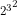(iv)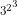(v) (0.008)(2 / 3)

(vi) (0.00243)(-3 / 5)

(vii)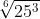(viii)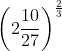Solution:

(i) 60 = 1

(ii) (1 / 2)-3 = (2)3

We get,

(1 / 2)-3 = 8

(iii)= 26

We get,

= 64(iv)= 36

We get,

= 729(v) (0.008)(2 / 3) = (0.23)(2 / 3)

= (0.2)3 × 2 / 3

= (0.2)2

We get,

(0.008)(2 / 3) = 0.04

(vi) (0.00243)(-3 / 5) = {1 / (0.00243)(3 / 5)}

= {1 / (0.35)(3 / 5)}

= {1 / (0.3)3}

We get,

(0.00243)(-3 / 5) = (1 / 0.027)

(vii)=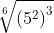=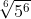= 56 × 1 / 6

We get,

= 5(viii)= (64 / 27)(2 / 3)

= (4 / 3)3 × 2 / 3

= (4 / 3)2

We get,= (16 / 9)

2. Evaluate the following:

(a) 94 ÷ 27(-2 / 3)

(b) 7-4 × (343)(2 / 3) ÷ (49)(- 1 / 2)

(c) (64 / 216)(2 / 3) × (16 / 36)(- 3 / 2)

Solution:

(a) 94 ÷ 27(-2 / 3) = {(3)2}4 ÷ {(3)3}(-2 / 3)

On further calculation, we get,

= (3)2 × 4 ÷ (3)3 × (- 2 / 3) [Using (am)n = amn]

= (3)8 ÷ (3)-2

= (3)8 – (-2) [Using am ÷ an = am – n]

= (3)8 + 2

= (3)10

This can be written as,

= (3)2 × 5

= {(3)2}5

= (9)5

We get,

= 59049

(b) 7-4 × (343)(2 / 3) ÷ (49)(- 1 / 2)

On calculating further, we get,

= 7-4 × (73)(2 / 3) ÷ (72)(- 1 / 2)

= 7-4 × 73 × 2 / 3 ÷ 72 × (- 1 / 2)

= 7-4 × 72 ÷ 7-1

= 7-4 + 2 – (-1) [Using am × an = am + n and am ÷ an = am – n]

= 7-4 + 2 + 1

We get,

= 7-1

= (1 / 7) [Using a-m = (1 / am)]

(c) (64 / 216)(2 / 3) × (16 / 36)(- 3 / 2)

On further calculation, we get,

= {(26)2 / 3 / (63)2 / 3} × {(24)-3 / 2 / (62)– 3 / 2}

= {(2)6 × 2 / 3 / (6)3 × 2 / 3} × {(2)4 × (-3 / 2) / (6)2 × (- 3 / 2)}

[Using (am)n = amn]

= {(2)2 × 2 / (6)2} × {(2)2 × (- 3) / (6)– 3}

= {(2)4 / (6)2} × {(2)-6 / (6)-3}

We get,

= {(2)4 / (6)2} × {(6)3 / (2)6}

[Using a-m = (1 / am)

= {(2)4 / (2)6} × {(6)3 / (6)2}

= (2)4 – 6 × (6)3 – 2

[Using am ÷ an = am – n]

= (2)-2 × (6)1

= (1 / 22) × 6

= (1 / 4) × 6

We get,

= (3 / 2)

3. Write each of the following in the simplest form:

(a) (a3)5 × a4

(b) a2 × a3 ÷ a4

(c) a1 / 3 ÷ a-2 / 3

(d) a-3 × a2 × a0

(e) (b-2 – a-2) ÷ (b-1 – a-1)

Solution:

(a) (a3)5 × a4 = (a)3 × 5 × a4

[Using (am)n = amn], we get,

= (a)15 × a4

= a15 + 4

[Using am × an = am + n]

We get,

= a19

(b) a2 × a3 ÷ a4 = a2 + 3 – 4

[Using am × an = am + n and am ÷ an = am – n]

We get,

= a1

= a

(c) a1 / 3 ÷ a-2 / 3 = a(1 / 3) – (- 2 / 3)

[Using am ÷ an = am – n]

We get,

= a(1 / 3) + (2 / 3)

= a(1 + 2) / 3

= a1

= 0

(d) a-3 × a2 × a0 = a-3 + 2 + 0

[Using am × an = am + n]

= a-1

We get,

= (1 / a)

(e) (b-2 – a-2) ÷ (b-1 – a-1)

This can be written as,

= (1/b2 – 1/a2) / (1/b – 1/a)

= {(1/b)2 – (1/a)2} / (1/b – 1/a)

= {(1/b + 1/a) (1/b – 1/a)} / (1/b – 1/a)

We get,

= (1/b + 1/a)

4. Evaluate the following:

(i) (23 × 35 × 242) / (122 × 183 × 27)

(ii) (43 × 37 × 56) / (58 × 27 × 33)

(iii) (122 × 75-2 × 35 × 400) / (482 × 15-3 × 525)

(iv) (26 × 5-4 × 3-3 × 42) / (83 × 15-3 × 25-1)

Solution:

(i) (23 × 35 × 242) / (122 × 183 × 27)

This can be written as,

= {23 × 35 × (23 × 3)2} / (22 × 3)2 × (2 × 32)3 × (33)

= (23 × 35 × 26 × 32) / (24 × 32 × 23 × 36 × 33)

= (29 × 37) / (27 × 311)

On further calculation, we get,

= (29 – 7 / 311 – 7)

= 22 / 34

We get,

= (4 / 81)

(ii) (43 × 37 × 56) / (58 × 27 × 33)

This can be written as,

= (22)3 × 37 – 3} / (58 – 6 × 27)

= (26 × 37 – 3) / (58 – 6 × 27)

On further calculation, we get,

= (26 × 34) / (52 × 27)

= {34 / (52 × 27 – 6)}

= {81 / (52 × 21)}

We get,

= (81 / 50)

(iii) (122 × 75-2 × 35 × 400) / (482 × 15-3 × 525)

This can be written as,

= (122 × 35 × 400 × 153) / (482 × 525 × 752)

= {(22 × 3)2 × (7 × 5) × (24 × 52) × (3 × 5)3} / {(24 × 3)2 × (3 × 52 × 7) × (3 × 52)2}

On calculating further, we get,

= (24 + 4 × 32+ 3 × 51 + 2 + 3 × 7) / (28 × 32 + 1 + 2 × 54 + 2 × 7)

= (28 × 35 × 56 × 7) / (28 × 35 × 56 × 7)

We get,

= 1

(iv) (26 × 5-4 × 3-3 × 42) / (83 × 15-3 × 25-1)

= (26 × 42 × 153 × 251) / (83 × 54 × 33)

This can be written as,

= {(26 × (22)2 × (3 × 5)3 × (52)1} / {(23)3 × 54 × 33}

= (26 + 4 × 33 × 53 + 2) / (29 × 33 × 54)

= (210 – 9 × 55 – 4)

= (2 × 5)

We get,

= 10

5. Simplify the following and express with positive index:

(a) 3p-2q3 ÷ 2p3q-2

(b) {(p-3)2 / 3}1 / 2

Solution:

(a) 3p-2q3 ÷ 2p3q-2

This can be written as,

= (3p-2q3) / (2p3q-2)

= (3 / 2) {(p-2 / p3) × (q3 / q-2)}

= (3 / 2) {(p-2 ÷ p3) × (q3 ÷ q-2)}

= (3 / 2) {(p– 2 – 3) × (q3 – (- 2))} [Using am ÷ an = am – n]

= (3 / 2) {(p-5) × (q5)}

= (3 / 2) {(1 / p5) × (q5)}

We get,

= (3q5 / 2p5)

(b) {(p-3)2 / 3}1 / 2

= p-3 × (2 / 3) × (1 / 2)

[Using (am)n = amn]

= p– 1

We get,

= (1 / p)

6. Evaluate the following:

(i) {1 – (15 / 64)}– 1 / 2

(ii) (8 / 27)– 2 / 3 – (1 / 3)– 2 – 70

(iii) 95 / 2 – 3 × 50 – (1 / 81) – 1 / 2

(iv) (27)2 / 3 × (8)– 1 / 6 ÷ (18)– 1 / 2

(v) (16)3 / 4 + 2 (1 / 2)-1 × 30

(vi)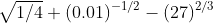Solution:

(i) {1 – (15 / 64)}– 1 / 2

On taking LCM, we get,

= {(64 – 15) / 64}– 1 / 2

= (49 / 64) – 1 / 2

= (64 / 49)1 / 2

We get,

= (8 / 7)

(ii) (8 / 27)– 2 / 3 – (1 / 3)– 2 – 70

= (27 / 8)2 / 3 – (1 / 3)-2 – 70

= (27 / 8)2 / 3 – (3)2 – 1

On further calculation, we get,

= (3 / 2)3 × 2 / 3 – 9 – 1

= (3 / 2)2 – 10

= (9 / 4) – 10

On taking LCM, we get,

= {(9 – 40) / 4}

= (- 31 / 4)

(iii) 95 / 2 – 3 × 50 – (1 / 81) – 1 / 2

On further calculation, we get,

= 32 × 5 / 2 – 3 × 1 – (81)1 / 2

= 35 – 3 – 92 × 1 / 2

= 243 – 3 – 9

We get,

= 231

(iv) (27)2 / 3 × (8)– 1 / 6 ÷ (18)– 1 / 2

This can be written as,

= 33 × 2 / 3 × {1 / (23 × 1 / 6)} ÷ (1 / 18)1 / 2

= (32) / (21 / 2) × (2 × 32)1 / 2

= (32 / 21 / 2) × 21 / 2 × 3

We get,

= 32 + 1

= 33

= 27

(v) (16)3 / 4 + 2 (1 / 2)-1 × 30

= 24 × 3 / 4 + 2 × 2 × 1

On further calculation, we get,

= 23 + 4

= 8 + 4

We get,

= 12

(vi)= (1 / 22)1 / 2 + (0.1)-1 – 32

= (1 / 2) + (0.1)-1 – 32

= (1 / 2) + (1 / 0.1) – 9

= (1 / 2) + (10 / 1) – 9

= (1 / 2) + 1

On taking LCM, we get,

= {(1 + 2) / 2}

= (3 / 2)

7. Simplify the following:

(a) (27x9)2 / 3

(b) (8x6y3)2 / 3

(c) (64a12 / 27b6)– 2 / 3

(d) (36m-4 / 49n-2)– 3 / 2

(e) (a1 / 3 + a– 1 / 3) (a2 / 3 – 1 + a– 2 / 3)

(f)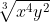÷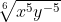(g) {(am){m – (1 / m)}}(1 / m + 1)

(h) xm + 2n. x3m – 8n ÷ x5m – 60

(i) (81)3 / 4 – (1 / 32)– 2 / 5 + 81 / 3. (1 / 2)-1. 20

(j) (27/343)2/3 ÷ {1/ (625/1296)1/4} x 536 /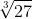Solution:

(a) (27x9)2 / 3

This can be written as,

= (33x9)2 / 3

= (33)2 / 3(x9)2 / 3 [Using (a × b)n = an × bn]

On calculating further,

We get,

= (3)3 × 2 / 3(x)9 × 2 / 3 [Using (am)n = amn]

= (3)2x3 × 2

We get,

= 9x6

(b) (8x6y3)2 / 3

This can be written as,

= (23x6y3)2 / 3

= (23)2 / 3 (x6)2 / 3(y3)2 / 3 [Using (a × b)n = an × bn]

= (2)3 × 2 / 3(x)6 × 2 / 3(y)3 × 2 / 3 [Using (am)n = amn]

= (2)2(x)2 × 2(y)2

We get,

= 4x4y2

(c) (64a12 / 27b6)– 2 / 3

This can be written as,

= {(26a12) / (33b6)}– 2 / 3

= {26 × (- 2 / 3)a12 × (- 2 / 3)} / {33 × (- 2 / 3)b6 × (- 2 / 3)}

[Using (a × b)n = an × bn and (a / b)n = (an / bn)]

On further calculation, we get,

= (2– 4a– 8) / (3– 2b– 4)

= (32b4) / (24a8) [Using a-n = (1 / an)]

We get,

= (9b4 / 16a8)

(d) (36m-4 / 49n-2)– 3 / 2

This can be written as,

= {(62m-4) / (72n– 2)}– 3 / 2

= {62 × (- 3 / 2) m-4 × (- 3 / 2)} / {72 × (- 3 / 2) n– 2 × (- 3 / 2)}

[Using (a × b)n = an × bn and (a / b)n = (an / bn)]

On further calculation, we get,

= (6-3 m6) / (7– 3n3)

= (73m6) / (63n3)

[Using a– 1 = (1 / an)]

We get,

= (343m6) / (216n3)

(e) (a1 / 3 + a– 1 / 3) (a2 / 3 – 1 + a– 2 / 3)

= a1 / 3(a2 / 3 – 1 + a– 2 / 3) + a– 1 / 3(a2 / 3 – 1 + a– 2 / 3)

On simplification, we get,

= (a1 / 3 × a2 / 3 – a1 / 3 × 1 + a1 / 3 × a– 2 / 3) + (a– 1 / 3 × a2 / 3 – a– 1 / 3 × 1 + a– 1 / 3 × a– 2 / 3)

= {a(1 / 3) + (2 / 3) – a1 / 3 × 1 + a(1 / 3) + (- 2 / 3)} + {a(- 1 / 3) + (2 / 3) – a– 1 / 3 × 1 + a(- 1 / 3) + (- 2 / 3)}

[Using am × an = am + n]

= {a1 – a1 / 3 + a– 1 / 3} + {a1 / 3 – a– 1 / 3 + a– 1}

= {a – a1 / 3 + a– 1 / 3 + a1 / 3 – a– 1 / 3 + (1 / a)}

We get,

= {a + (1 / a)}

(f)÷This can be written as,

= (x4y2)1 / 3 ÷ (x5y– 5)1 / 6

On calculating further, we get,

= {x4 × (1 / 3) y2 × (1 / 3)} ÷ {x5 × (1 / 6) y– 5 × (1 / 6)}

[Using (am)n = amn]

= {x(4 / 3) y(2 / 3)} ÷ {x(5 / 6) y(- 5 / 6)}

= {x(4 / 3)y(2 / 3)} / {x(5 / 6) y(- 5 / 6)}

= x(4 / 3) – (5 / 6) y(2 / 3) – (- 5 / 6) [Using am ÷ an = am – n]

= x(1 / 2) y(3 / 2)

= x(1 / 2) (y3)(1 / 2)

[Using (am)n = amn]

= x y3

We get,

=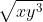(g) {(am){m – (1 / m)}}(1 / m + 1)

= (a) m × {m – (1 / m)} × {1 / (m + 1)}

[Using (am)n = amn]

Now,

Consider,

m × {m – (1 / m)} × {1 / (m + 1)}

= (m2 – 1) × {1 / (m + 1)}

= m2 × {1 / (m + 1)} – 1 × {1 / (m + 1)}

= {m2 / (m + 1)} – {1 / (m + 1)}

= {(m2 – 1)} / {(m + 1)}

= {(m – 1) (m + 1)} / (m + 1)

We get,

= (m – 1)

Therefore, (a) m × {m – (1 / m)} × {1 / (m + 1)} = am – 1

(h) xm + 2n. x3m – 8n ÷ x5m – 60

= xm + 2n + 3m – 8n – 5m – (- 60)

[Using am × an = am + n and am ÷ an = am – n]

= xm + 2n + 3m – 8n – 5m + 60

We get,

= x– m – 6n + 60

(i) (81)3 / 4 – (1 / 32)– 2 / 5 + 81 / 3. (1 / 2)-1. 20

This can be written as,

= (34)(3 / 4) – (1 / 25)(- 2 / 5) + (23)(1 / 3). (1 / 2)– 1 × 1

[Using a0 = 1]

= 34 × (3 / 4) – {1 / 25 × (- 2 / 5)} + 23 × (1 / 3). (2)1

= 33 – {1 / 2– 2} + 21. (2)1

= 33 – 22 + 2(1 + 1)

[Using am × an = am + n and a– n = (1 / an)]

= 33 – 22 + 22

We get,

= 33

= 27

(j) (27/343)2/3 ÷ {1/ (625/1296)1/4} x 536 /This can be written as,

= {33 / (73}2/3 ÷ [1/{54 / (24 x 34)}1/4] x (23 x 67) /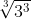= (33/ 73)2/3 ÷ [1/ {54 / (24 x 34)}1/4] x (23 x 67) / (33)1/3

= (33 x 2/3 / 73 x 2/3) ÷ [1/ {54 x ¼ / (24 x ¼ x 34 x ¼)] x (23 x 67) / (33 x 1/ 3)

Using (am)n = am n

= (32 / 72) ÷ [1 / {51 / (21 x 31)}] x (23 x 67) / 31

= (32 / 72) ÷ {(21 x 31) / 51} x (23 x 67) / 31

= (32 / 72) x {51 / (21 x 31)} x (23 x 67) / 31

On further calculation, we get,

= 32 – 1 – 1 x 23 – 1 x 51 x 7-2 x 67

Using a am ÷ an = am – n

= 30 x 22 x 51 x 7-2 x 67

= 1 x 4 x 51x 7-2 x 67

= 1340 / 49

= 27.34

8. Simplify the following:

(i) (5x × 7 – 5x) / (5x + 2 – 5x + 1)

(ii) (3x + 1 + 3x) / (3x + 3 – 3x + 1)

(iii) (2m × 3 – 2m) / (2m + 4 – 2m + 1)

(iv) (5n + 2 – 6.5n + 1) / (13. 5n – 2. 5n + 1)

Solution:

(i) (5x × 7 – 5x) / (5x + 2 – 5x + 1)

On taking common terms, we get,

= {5x (7 – 1)} / {5x + 1 (5 – 1)}

= (5x – x – 1 × 6) / 4 [Using am ÷ an = am – n]

= (5– 1 × 6) / 4

= {6 / (5 × 4)}

We get,

= (3 / 10)

(ii) (3x + 1 + 3x) / (3x + 3 – 3x + 1)

On taking common terms, we get,

= {3x (3 + 1)} / {3x (33 – 3)}

= {4 / (27 – 3)}

= (4 / 24)

We get,

= (1 / 6)

(iii) (2m × 3 – 2m) / (2m + 4 – 2m + 1)

On taking common terms, we get,

= {2m (3 – 1)} / {2m (24 – 2)}

= {2 / (16 – 2)}

= (2 / 14)

We get,

= (1 / 7)

(iv) (5n + 2 – 6.5n + 1) / (13. 5n – 2. 5n + 1)

On taking common terms, we get,

= {5n (52 – 6 × 5)} / {5n (13 – 2 × 5)}

= (25 – 30) / (13 – 10)

We get,

= (- 5 / 3)

9. Solve for x:

(a) 22x + 1 = 8

(b) 3 × 7x = 7 × 3x

(c) 2x + 3 + 2x + 1 = 320

(d) 9 × 3x = (27)2x – 5

(e) 22x + 3 – 9 × 2x + 1 = 0

(f) 1 = px

(g) p3 × p– 2 = px

(h) p– 5 = (1 / px + 1)

(i) 22x + 2x + 2 – 4 × 23 = 0

(j) 9 x 81x = 1/ 27x – 3

(k) 22x – 1 – 9 × 2x – 2 + 1 = 0

(l)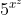: 5x = 25: 1

(m)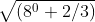= (0.6)2 – 3x

(n)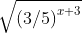= (27– 1) / (125– 1)

(o) 9x + 4 = 32 × (27)x + 1

Solution:

(a) 22x + 1 = 8

This can be written as,

22x + 1 = 23

2x + 1 = 3

2x = 3 – 1

2x = 2

We get,

x = 1

(b) 3 × 7x = 7 × 3x

(7x / 7) = (3x / 3)

7x – 1 = 3x – 1 [Using am ÷ an = am – n]

7x – 1= 3x – 1 × 1

7x – 1 = 3x – 1 × 70

[Using a0 = 1]

x – 1 = 0

We get,

x = 1

(c) 2x + 3 + 2x + 1 = 320

This can be written as,

2x + 3 + 2x + 1 = 26 × 5

2x. 23 + 2x.21 = 26 × 5

On taking common terms, we get,

2x (23 + 21) = 26 × 5

2x (8 + 2) = 26 × 5

2x (10) = 26 × 5

2x (10 / 5) = 26

2x. 2 = 26

(2x.2) / 26 = 1

2x + 1 – 6 = 1 × 20

2x – 5 = 1 × 20

x – 5 = 0

We get,

x = 5

(d) 9 × 3x = (27)2x – 5

This can be written as,

32 × 3x = (33)2x – 5

32 × 3x = 33 × (2x – 5)

On further calculation, we get,

32 + x = 36x – 15

1 = (36x – 15) / (32 + x)

1 = 36x – 15 – 2 – x

30 = 35x – 17

5x – 17 = 0

5x = 17

We get,

x = (17 / 5)

(e) 22x + 3 – 9 × 2x + 1 = 0

This can be written as,

22x.23 – 9 × 2x + 1 = 0

Put 2x = t

Then,

22x = t2

So,

22x.23 – 9 × 2x + 1 = 0 becomes,

8t2 – 9t + 1 = 0

8t2 – 8t – 1t + 1 = 0

On taking common terms, we get,

8t (t – 1) – 1 (t – 1) = 0

(t – 1) = 0 or (8t – 1) = 0

t = 1 or t = (1 / 8)

2x = 1 or 2x = (1 / 23)

2x = 20 or 2x = 2-3

Hence,

x = 0 or x = – 3

(f) 1 = px

p0 = px [Using a0 = 1]

Therefore,

x = 0

(g) p3 × p– 2 = px

p3 + (- 2) = px [Using am × an = am + n]

p3 – 2 = px

p1 = px

Hence,

x = 1

(h) p– 5 = (1 / px + 1)

p-5 × px + 1 = 1

p– 5 + x + 1 = 1 [Using am × an = am + n]

px – 4 = p0

x – 4 = 0

We get,

x = 4

(i) 22x + 2x + 2 – 4 × 23 = 0

This can be written as,

22x + 2x + 2 – 22 × 23 = 0

22x + 2x.22 – 22 + 3 = 0 [Using am × an = am + n]

22x + 2x.22 – 25 = 0

22x + 2x. 4 – 32 = 0

Put 2x = t

Then, 22x = t2

22x + 2x.4 – 32 = 0 becomes,

t2 + 4t – 32 = 0

t2 + 8t – 4t – 32 = 0

On taking common terms, we get,

t (t + 8) – 4 (t + 8) = 0

t + 8 = 0 or t – 4 = 0

t = – 8 or t = 4

2x = – 8 or 2x = 4

2x = – 23 or 2x = 22

Now,

Consider second equation,

2x = 22

We get,

x = 2

(j) 9 x 81x = 1/ 27x – 3

This can be written as,

32 x 34x = 1/ 33 (x – 3)

32 x 34x = 1/ 33x – 9

Using (am)n = amn

32 x 34x x 33x – 9 = 1

32 + 4x + 3x – 9 = 1 x 30

On further calculation, we get,

2 + 4x + 3x – 9 = 0

7x – 7 = 0

7x = 7

x = 1

(k) 22x – 1 – 9 × 2x – 2 + 1 = 0

This can be written as,

22x. 2-1 – 9 × 2x. 2– 2 + 1 = 0

Let 2x = t,

So, 22x = t2

Then,

22x.2– 1 – 9 × 2x.2– 2 + 1 = 0 becomes,

(t2 / 2) – 9 × (t / 22) + 1 = 0

(t2 / 2) – (9t / 4) + 1 = 0

Taking LCM, we get,

2t2 – 9t + 4 = 0

2t2 – 8t – t + 4 = 0

2t (t – 4) – 1 (t – 4) = 0

t – 4 = 0 or 2t – 1 = 0

t = 4 or t = (1 / 2)

Hence,

2x = 4 or 2x = (1 / 2)

2x = 22 or 2x = 2– 1

Therefore,

x = 2 or x = – 1

(l): 5x = 25: 1

This can be written as,

(/ 5x) = (25 / 1)

(/ 5x) = (52 / 1)

= 52 × 5x= 52 + xx2 = 2 + x

x2 – x – 2 = 0

(x – 2) (x + 1) = 0

x – 2 = 0 or x + 1 = 0

Therefore,

x = 2 or x = – 1

(m)= (0.6)2 – 3x

This can be written as,

{1 + (2 / 3)}1 / 2 = (6 / 10)2 – 3x

On taking LCM, we get,

(5 / 3)1 / 2 = (3 / 5)2 – 3x

(3 / 5)– 1 / 2 = (3 / 5)2 – 3x

(- 1 / 2) = 2 – 3x

-1 = 2 (2 – 3x)

– 1 = 4 – 6x

– 1 – 4 = – 6x

– 5 = – 6x

Hence,

x = (5 / 6)

(n)= (27– 1) / (125– 1)

This can be written as,

(3 / 5)(x + 3) × (1 / 2) = {(33)-1 / (53)-1}

(3 / 5)(x + 3) / 2 = (3 / 5)– 3

(x + 3) / 2 = – 3

x + 3 = – 3 × 2

x + 3 = – 6

x = – 6 – 3

We get,

x = – 9

(o) 9x + 4 = 32 × (27)x + 1

This can be written as,

9x + 4 = 32 × (33)x + 1

32 (x + 4) = 32 × 33x + 3

32x + 8 = 32 + 3x + 3

Hence,

2x + 8 = 2 + 3x + 3

2x + 8 = 3x + 5

3x – 2x = 8 – 5

We get,

x = 3

10. Find the value of k in each of the following:

(i)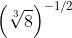= 2k

(ii)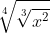= xk

(iii)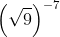×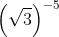= 3k

(iv) (1 / 3)– 4 ÷ 9 (- 1 / 3) = 3k

Solution:

(i)= 2k

This can be written as,

8(1 / 3) × (- 1 / 2) = 2k

(23)(1 / 3) × (- 1 / 2) = 2k

2(- 1 / 2) = 2k

Therefore,

k = (- 1 / 2)

(ii)= xk

This can be written as,

{(x2)1 / 3}1 / 4 = xk

On further calculation, we get,

(x2)1 / 12 = xk

x(2 / 12) = xk

x(1 / 6) = xk

Hence,

k = (1 / 6)

(iii)×= 3k

This can be written as,

{(32)(1 / 2)}– 7 × {(3)(1 / 2)}– 5 = 3k

3– 7 × 3(- 5 / 2) = 3k

3-7 – 5 / 2 = 3k

3(- 14 – 5) / 2 = 3k

3(- 19 / 2) = 3k

Therefore,

k = (- 19 / 2)

(iv) (1 / 3)– 4 ÷ 9 (- 1 / 3) = 3k

This can be written as,

(3– 1)– 4 ÷ (32)– 1 / 3 = 3k

34 ÷ 3(- 2 / 3) = 3k

34 – (- 2 / 3) = 3k

34 + 2 / 3 = 3k

3(14 / 3) = 3k

We get,

k = (14 / 3)

11. If a = 2(1 / 3) – 2(- 1 / 3), prove that 2a3 + 6a = 3

Solution:

Given

a = 2(1 / 3) – 2(- 1 / 3)

This can be written as,

a = 2(1 / 3) – {1 / 2(1 / 3)}

On taking cube on both sides, we get,

a3 = [2(1 / 3) – {1 / 2(1 / 3)}]3

On further calculation, we get,

a3 = 2 – (1 / 2) – 3 [2(1 / 3) – {1 / 2(1 / 3)}]

a3 = {(4 – 1) / 2} – 3a

a3 = (3 / 2) – 3a

We get,

2a3 + 6a = 3

12. If x = 3(2 / 3) + 3(1 / 3), prove that x3 – 9x – 12 = 0

Solution:

Given

x = 32 / 3 + 31 / 3

x3 = 32 + 3 + 3 × 32 / 3 × 31 / 3 (32 / 3 + 31 / 3)

x3 = 9 + 3 + 3 × 32 / 3 + 1 / 3 (x)

x3 = 12 + 9x

We get,

x3 – 9x – 12 = 0

13. If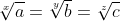and abc = 1, prove that x + y + z = 0

Solution:

Let= k

So,

a1 / x = k, b1 / y = k, c1 / z = k

We get,

a = kx, b = ky , c = kz

Also, given that,

abc = 1

kx × ky × kz = 1

kx + y + z = k0

We get,

x + y + z = 0

14. If ax = by = cz and b2 = ac, prove that y = 2xz / (z + x).

Solution:

Let ax = by = cz = k

So,

a = k1 / x, b = k1 / y, c = k1 / z

Also given that,

b2 = ac

k2 / y = k1 / x × k1 / z

k2 / y = k1 / x + 1 / z

(2 / y) = (1 / x) + (1 / z)

(2 / y) = (z + x) / zx

We get,

y = {2zx / (z + x)}

15. Show that: {1 / (1 + ap – q)} + {1 / (1 + aq – p)} = 1

Solution:

Consider LHS of the equation, i.e,

{1 / (1 + ap – q)} + {1 / (1 + aq – p)}

On taking LCM, we get,

= {(1 + aq – p) + (1 + ap – q)} / (1 + ap – q) (1 + aq – p)

= (2 + a– (p – q) + ap – q) / (1 + ap – q) (1 + a– (p – q))

= 2 + a– (p – q) + ap – q / (1 + a– (p – q) + ap – q + ap – q. a– (p – q))

= 2 + a– (p – q) + ap – q / (1 + a– (p – q) + ap – q + ap – q – p + q

= 2 + a– (p – q) + ap – q / (1 + a– (p – q) + ap – q + a0)

= 2 + a– (p – q) + ap – q / (1 + a– (p – q) + ap – q + 1)

= 2 + a– (p – q) + ap – q / (2 + a– (p – q) + ap – q)

We get,

= 1

= RHS

Therefore,

LHS = RHS

Hence, proved